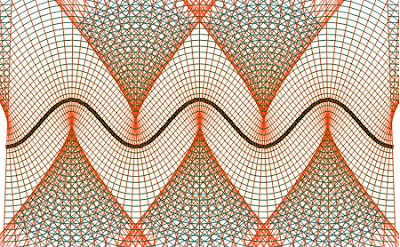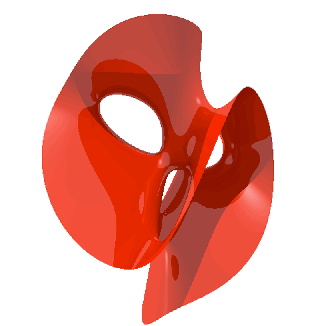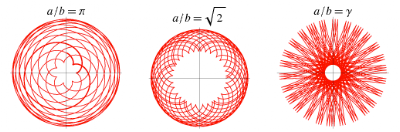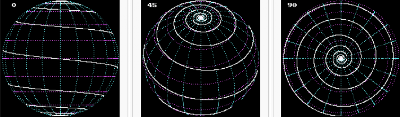## Friday, February 18, 2011

Geometry of Circles by Phillip Glass

## Thursday, February 17, 2011http://demonstrations.wolfram.com/CosineOffsetCurves/Hyperbolic geometry differs greatly from what we are used to in that the longest path between two points is a straight line. You can think of hyperbolic space like Euclidean space, but with a different way of defining the distance between two points (we call this a metric). Above is the hyperbolic equivalent of an icosidodecahedron. Check out more hyperbolic tilings here.

## Sunday, February 13, 2011

Clebsch Diagonal CubicThe surface is given by the equation x^3 + y^3 + z^3 + w^3 + t^3 = 0, restricted to the values x, y, z, w, t, such that x + y + z + w + t = 0.

## Monday, February 7, 2011Above are hypocycloids, curves produced by fixing a point on the circumference of a small circle of radius b, rolling around the inside of a large circle of radius a > b. If a/b is rational, i.e. it can be expressed as a fraction, the path will return to it's starting point. If the ratio is irrational, the path will never touch the same spot on the circumference of the larger circle more than once, and images such as the ones above result.The first few prime numbers represented in order from left to right in binary.

## Tuesday, February 1, 2011A mathematical structure known as a Lie algebra, E8 is one of the most complicated Lie algebras, and is used in string theory.A rhumb line (or loxodrome) runs from the north to the south pole passing through the intersections of the longitude and meridian at the same angle every time.
Einstein Cross: Gravity bends light so that two galaxies look like 5!Relativity predicts that gravity warps the shape of space and time so that light will no longer move along a straight path. One of the earliest confirmations of the theory was during a total eclipse which allowed us to see a star that wasn't quite in the right place. The sun's gravitational force bent the light rays so that the star appeared about an inch away from where it actually was.
In the image above, the galaxy in the center bends the light waves coming off of the object behind it so that we see 4 different images of the same object. This is called gravitational lensing.